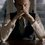# Some non-mathematical puzzles!!! Use your mind!!!

Prove the following just by drawing a single line:

5 + 5 + 5 = 550 [You cannot use the line on equality.]


THE EASY ONE - Use a number 3 times and addition sign(s) to get the number 60. You cannot use 20.

Another EASY ONE - Use the following only once to make an equation-

2, 3, 4, 5, = , +.Note by Kartik Sharma
7 years ago

This discussion board is a place to discuss our Daily Challenges and the math and science related to those challenges. Explanations are more than just a solution — they should explain the steps and thinking strategies that you used to obtain the solution. Comments should further the discussion of math and science.

When posting on Brilliant:

• Use the emojis to react to an explanation, whether you're congratulating a job well done , or just really confused .
• Ask specific questions about the challenge or the steps in somebody's explanation. Well-posed questions can add a lot to the discussion, but posting "I don't understand!" doesn't help anyone.
• Try to contribute something new to the discussion, whether it is an extension, generalization or other idea related to the challenge.

MarkdownAppears as
*italics* or _italics_ italics
**bold** or __bold__ bold
- bulleted- list
• bulleted
• list
1. numbered2. list
1. numbered
2. list
Note: you must add a full line of space before and after lists for them to show up correctly
paragraph 1paragraph 2

paragraph 1

paragraph 2

[example link](https://brilliant.org)example link
> This is a quote
This is a quote
    # I indented these lines
# 4 spaces, and now they show
# up as a code block.

print "hello world"
# I indented these lines
# 4 spaces, and now they show
# up as a code block.

print "hello world"
MathAppears as
Remember to wrap math in $$ ... $$ or $ ... $ to ensure proper formatting.
2 \times 3 $2 \times 3$
2^{34} $2^{34}$
a_{i-1} $a_{i-1}$
\frac{2}{3} $\frac{2}{3}$
\sqrt{2} $\sqrt{2}$
\sum_{i=1}^3 $\sum_{i=1}^3$
\sin \theta $\sin \theta$
\boxed{123} $\boxed{123}$

Sort by:

Answers will be posted on 1st August!!

- 7 years ago

Ah! You solved them all. So, no answers I can post today!! ;)

- 6 years, 12 months ago

Not sure if they the correct answer,

Question 2 :, using $5$ three times, $.$ $55+5=60$ $.$$.$ Question 1 : draw the line on the equal sign, consequently the equation becomes an inequality $.$ $5+5+5\neq550$

- 7 years ago

oh, didn't I say that you cannot use that inequality sign?

- 6 years, 12 months ago

You said nothing, you only told us to draw some line and he drew the line on the equality sign. There's nothing wrong with that, so if you don't like this solution, you'll have to change the problem a bit (add some constraints, e.g. 'you can't use the line on the equality sign').

- 6 years, 12 months ago

- 6 years, 12 months ago

by the way, solution to Q2 is correct!!!

- 6 years, 12 months ago

Question 3:

4+5=3²

Question 1: Is this possible?

5 + 5 4 5 = 550 (draw a line through the plus sign to make it a four)

- 7 years ago

or $2+5=3+4$

- 6 years, 12 months ago

But I think that you can only use the addition sign once.

- 6 years, 12 months ago

yeah. sorry, missed where he said 'only once'.

- 6 years, 12 months ago

Yeah, that's correct!!!

- 6 years, 12 months ago

Question 1

$5 + 545 = 550$

Question 2

$5 + 55 = 60$

Question 3

$4 + 5 = 3^2$

Am I right?

- 6 years, 12 months ago

solution to question 3 should be 4 + 5 = 3^2

otherwise you are absolutely correct!!!

You cannot use even "+" sign more than once!!!

- 6 years, 12 months ago

Oh, then what you said.

- 6 years, 12 months ago

sorry?

- 6 years, 12 months ago